Courses

# Chapter Notes - Decimals Class 6 Notes | EduRev

## Class 6 : Chapter Notes - Decimals Class 6 Notes | EduRev

The document Chapter Notes - Decimals Class 6 Notes | EduRev is a part of the Class 6 Course Mathematics (Maths) Class 6.
All you need of Class 6 at this link: Class 6

8. Decimals

Comparing Decimals: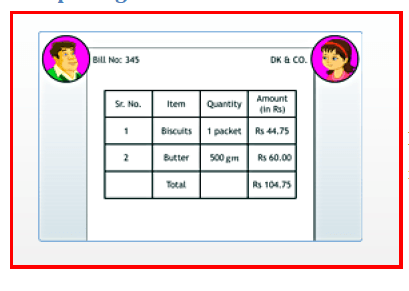Every fraction whose denominator is 10 can be written in decimal notation.

If a block of one unit is divided into 10 equal parts, then each part is 1/10 (one - tenth) of the unit. It is written as in decimal representation. The dot denotes the decimal point. Every fraction whose denominator is 10 can be written in decimal notation.

Eg: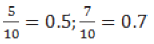If a block of one unit is divided into 100 equal parts, then each part is of the unit. It is written as in decimal notation. Every fraction whose denominator 100 can be written in decimal notation.

Eg: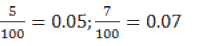To read decimals, we can use the following chart. The first digit to the right after the decimal point represents the tenths parts, the second the hundredths parts, and so on.

 Decimal point Tenths Hundredths Thousandths 1/10  = 0.1 1/100 = 0.01 1/1000 = 0.001

All decimal numbers can be represented on the number line. Every decimal number can be represented as a fraction. Any two decimal numbers can be compared. The comparison starts with the whole part of the numbers. If the whole parts are equal, then the tenth parts can be compared, and so on. Decimal numbers are used in many ways in real life. For example, in representing the units of money, length and weight, we use decimal numbers.

Page 38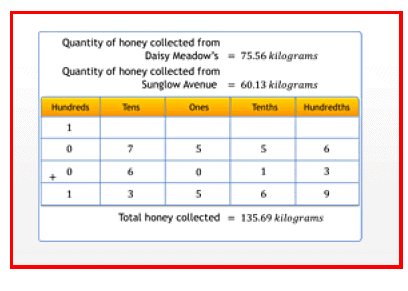To add or subtract decimal numbers, make sure that the decimal points of the given numbers are placed exactly one below another.

To add or subtract decimal numbers, make sure that the decimal points of the given numbers are placed exactly one below another. While adding or subtracting two decimal numbers, the number of digits after the decimal point should be equal. In case they are not equal, the gaps must be filled with zeros after the last digit.

For example:

1. To add 6.82 and 5

First insert zeros in the empty places after the decimal point so that both the numbers have the same number of digits after the decimal point. Next, write the numbers such that their decimal points are one below another.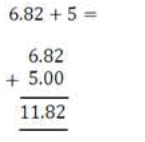2. To subtract 5 from 6.82

First insert zeros in the empty places after the decimal point so that both the numbers have the same number of digits after the decimal point. Next, write the numbers such that their decimal points are one below another.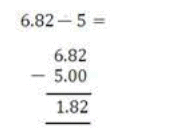Page 39

Addition or subtraction should be carried out from the extreme right side. Place the decimal point correctly after performing the addition or subtraction.

Offer running on EduRev: Apply code STAYHOME200 to get INR 200 off on our premium plan EduRev Infinity!

## Mathematics (Maths) Class 6

185 videos|229 docs|43 tests

,

,

,

,

,

,

,

,

,

,

,

,

,

,

,

,

,

,

,

,

,

;